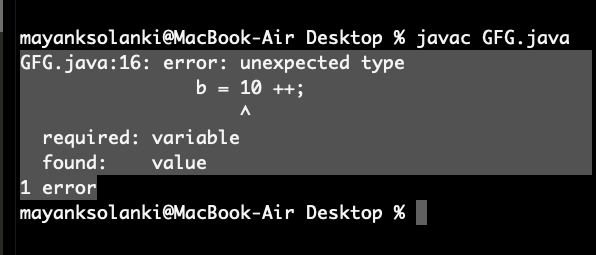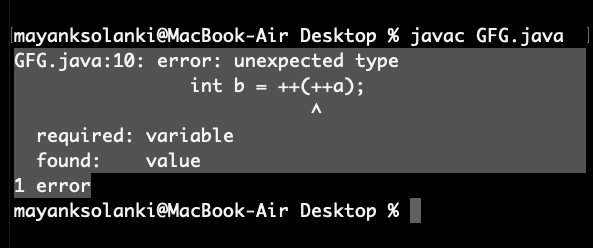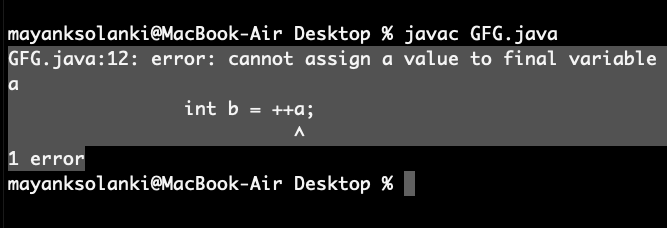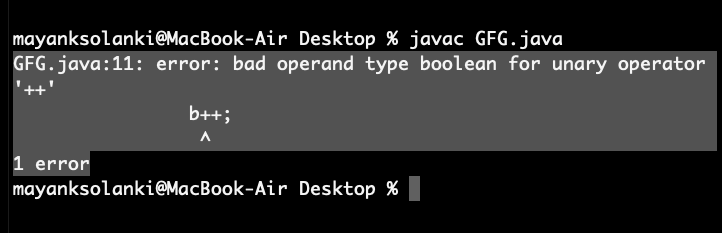# Interesting facts about Increment and Decrement operators in Java

• Difficulty Level : Easy
• Last Updated : 15 Oct, 2021

Increment operator is used to incrementing a value by 1. There are two varieties of increment operator:

• Post-Increment: Value is first used for computing the result and then incremented.
• Pre-Increment: Value is incremented first and then the result is computed.

Decrement operator is used for decrementing the value by 1. There are two varieties of decrement operators.

Attention reader! Don’t stop learning now. Get hold of all the important Java Foundation and Collections concepts with the Fundamentals of Java and Java Collections Course at a student-friendly price and become industry ready. To complete your preparation from learning a language to DS Algo and many more,  please refer Complete Interview Preparation Course.

• Post-decrement: Value is first used for computing the result and then decremented.
• Pre-decrement: Value is decremented first and then the result is computed.

Now let us do Interesting facts about Increment and Decrement operators:

• Can only be applied to variables only
• Nesting of both operators is not allowed
• They are not operated over final variables
• Increment and Decrement Operators can not be applied to boolean.

Let us discuss these 4 facts as listed above and do implement them as follows:

Fact 1: Can be applied to variables only

We can apply ++ and — operator only for variables but not for the constant values. If we are trying to apply ++ and — operator on the constant value then we will get a compile-time error which we will be seeing in example 1B after below example as follows:

Example 1:

## Java

 `// Java program to illustrate Increment``// and Decrement Operators``// Can be Applied to Variables Only` `// Main class``public` `class` `GFG {``  ` `    ``// main driver method``    ``public` `static` `void` `main(String[] args)``    ``{``      ` `        ``int` `a = ``10``;``        ``int` `b = ++a;``       ` `       ``// Printing value inside variable``        ``System.out.println(b);``    ``}``}`
Output
`11`

Example 2:

## Java

 `// Java program to Illustrate Increment and Decrement``// operators Can be applied to variables only` `// Main class``public` `class` `GFG {` `    ``// main driver method``    ``public` `static` `void` `main(String[] args)``    ``{``        ``// Declaring and initializing variable``        ``int` `a = ``10``;` `        ``int` `b = ++a;` `        ``// This is change made in above program``        ``// which reflects error during compilation``        ``b = ``10` `++;` `        ``// Printing its value``        ``System.out.println(b);``    ``}``}`

Output:Fact 2: Nesting of both ++ and — operators are not allowed

Example

## Java

 `// Java program to Illustrate Nesting Can Not be Applied``// to Increment and Decrement Operators` `// Main class``public` `class` `GFG {` `    ``// Main driver method``    ``public` `static` `void` `main(String[] args)``    ``{``        ``int` `a = ``10``;``        ``int` `b = ++(++a);` `        ``// Printing the value inside variable``        ``System.out.println(b);``    ``}``}`

Output:Fact 3: Final variables can’t apply increment and decrement operator

The increment and decrement operators can not be applied to final variables because of the simple reason that their value can not be changed.

Example

## Java

 `// Java Program to Illustrate Increment and Decrement``// Operators Can not be applied to final variables` `// Main class``public` `class` `GFG {` `    ``// MAin driver method``    ``public` `static` `void` `main(String[] args)``    ``{``        ``// Declaring and initializing final variable``        ``final` `int` `a = ``10``;` `        ``int` `b = ++a;` `        ``// Trying to print the updated value inside variable``        ``System.out.println(b);``    ``}``}`

Output:Fact 4: Increment and Decrement Operators can not be applied to boolean

We can apply ++ and — operators for all primitive data types except Boolean type as it only has true and false which even sounds impractical.

Example

## Java

 `// Java program to Illustrate Increment and Decrement``// Operators Can not be applied boolean data type` `// Main class``public` `class` `GFG {` `    ``// Main driver method``    ``public` `static` `void` `main(String[] args)``    ``{``        ``// Initially declaring boolean as false``        ``boolean` `b = ``false``;``        ``b++;` `        ``// Trying printing the bool value``        ``System.out.println(b);``    ``}``}`

Output: